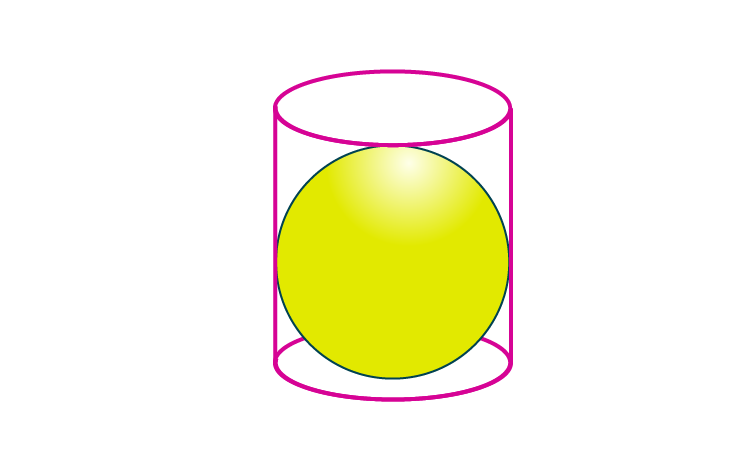Newbie

# A cylindrical can whose base is horizontal and of radius 3.5 cm contains sufficient water so that when a sphere is placed in the can, the water just covers the sphere. Given that the sphere just fits into the can, calculate : (i) the total surface area of the can in contact with water when the sphere is in it. (ii) the depth of the water in the can before the sphere was put into the can. Given your answer as proper fractions.

• 2

An Important Question of class 10 Based on Mensuration Chapter of M.L Aggarwal for ICSE BOARD.
A cylindrical can whose base is horizontal and its radius is given it contains sufficient water so that when a sphere is placed in the can, the water just covers the sphere.
Given that the sphere just fits into the can,
So calculate the total surface area of the can in contact with water when the sphere is in it and the depth of the water in the can before the sphere was put into the can. Given your answer as proper fractions.
This is the Question Number 29, Exercise 17.5 of M.L Aggarwal.

Share

1.(i)Given radius of the cylinder, r = 3.5 cm

Diameter of the sphere = height of the cylinder

= 3.5×2

= 7 cm

So radius of sphere, r = 7/2 = 3.5 cm

Height of cylinder, h = 7 cm

Total surface area of can in contact with water = curved surface area of cylinder+base area of cylinder.

= 2rh+r2

= r(2h+r)

= 22/7)×3.5×(2×7+3.5)

= (22/7)×3.5×(14+3.5)

= 11×17.5

= 192.5 cm2

Hence the surface area of can in contact with water is 192.5 cm2.

(ii)Let the depth of the water before the sphere was put be d.

Volume of cylindrical can = volume of sphere+ volume of water

r2h = (4/3)r3+r2d

r2h = r2{(4/3)r +d)}

h = (4/3)r +d

d = h – (4/3)r

d = 7 – (4/3)×3.5

d = (21-14)/3

d = 7/3

Hence the depth of the water before the sphere was put into the can is 7/3 cm.

• 1